| Next|| Previous || Top |

# Transport in Dual Porosity Media

Water in structured soils and in solid rock has often a dual character with regard to flow: part of the water is mobile and flows along the conduits (continuous joints, fractures, connected porosity), while another part remains immobile or stagnant within the structural units. Exchange of water and solutes between the two parts may occur through diffusion. Dual porosity media can be simulated in PHREEQC either with the first-order exchange approximation or with finite differences for diffusion in the stagnant zone.

## First-Order Exchange Approximation

Diffusive exchange between mobile and immobile water can be formulated in terms of a mixing process between mobile and stagnant cells. In the following derivation, one stagnant cell is associated with one mobile cell. The first-order rate expression for diffusive exchange is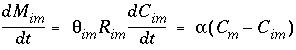, (119)

where subscript m indicates mobile and im indicates immobile, M im are moles of chemical in the immobile zone,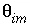is porosity of the stagnant (immobile) zone (a fraction of total volume, unitless), R im is retardation in the stagnant zone (unitless), C im is the concentration in stagnant water (mol/kgw), t is time (s), C m is the concentration in mobile water (mol/kgw), and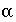is the exchange factor (s -1 ). The retardation is equal to R = 1 + dq / dC, which is calculated implicitly by PHREEQC through the geochemical reactions. The retardation contains the change dq in concentration of the chemical in the solid due to all chemical processes including exchange, surface complexation, kinetic and mineral reactions; it may be non-linear with solute concentration and it may vary over time for the same concentration.

The equation can be integrated with the following initial conditions: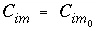and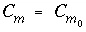, at t = 0, and by using the mole-balance condition:.

The integrated form of equation 119 is then: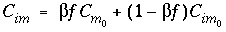, (120)

where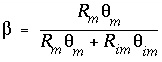,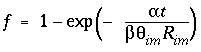,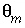is the water filled porosity of the mobile part (a fraction of total volume, unitless), and R m is the retardation in the mobile area.

A mixing factor, mixf im , can be defined that is a constant for a given time t :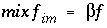. (121)

When mixf im is entered in equation 120, the first-order exchange is shown to be a simple mixing process in which fractions of two solutions mix: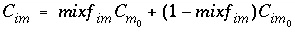. (122)

Similarly, an equivalent mixing factor, mixf m , for the mobile zone concentrations is obtained with the mole-balance equation: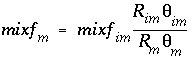(123)

and the concentration of C m at time t is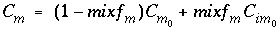. (124)

The exchange factorcan be related to specific geometries of the stagnant zone (Van Genuchten, 1985). For example, when the geometry is spherical, the relation is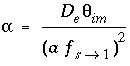, (125)

where D e is the diffusion coefficient in the sphere (m 2 /s), a is the radius of the sphere (m), and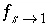is a shape factor for sphere-to-first-order-model conversion (unitless). Other geometries can likewise be transformed to a value forusing other shape factors (Van Genuchten, 1985). These shape factors are given in table 1.

Table 1. --Shape factors for diffusive first-order exchange between cells with mobile and immobile water

Shape of
stagnant region

Dimensions
(x, y, z) or
(2r, z)

First-order
equivalent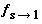Sphere

2 a

0.210

2 a = diameter

Plane sheet

2 a ,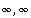0.533

2 a = thickness

Rectangular prism

2 a , 2 a ,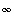0.312

rectangle

2 a , 2 a , 16 a

0.298

2 a , 2 a , 8 a

0.285

2 a , 2 a , 6 a

0.277

2 a , 2 a , 4 a

0.261

2 a , 2 a , 3 a

0.246

2 a , 2 a , 2 a

0.220

cube 2 a x2 a x2 a

2 a , 2 a , 4 a /3

0.187

2 a , 2 a , a

0.162

2 a , 2 a , 2 a /3

0.126

2 a , 2 a , 2 a /4

0.103

2 a , 2 a , 2 a /6

0.0748

2 a , 2 a , 2 a /8

0.0586

Solid cylinder

2 a ,0.302

2 a = diameter

2 a , 16 a

0.289

2 a , 8 a

0.277

2 a , 6 a

0.270

2 a , 4 a

0.255

2 a , 3 a

0.241

2 a , 2 a

0.216

2 a , 4 a /3

0.185

2 a , a

0.161

2 a , 2 a /3

0.126

2 a , 2 a /4

0.103

2 a , 2 a /6

0.0747

2 a , 2 a /8

0.0585

Pipe wall

2 r i , 2 r o ,2 r i = pore diameter

(surrounds the

2 a , 4 a

0.657

2 r o = outer diameter of pipe (Enter wall thickness r o - r i = a in Equation 125).

mobile pore)

2 a , 10 a

0.838

2 a , 20 a

0.976

2 a , 40 a

1.11

2 a , 100 a

1.28

2 a , 200 a

1.40

2 a , 400 a

1.51

An analytical solution is known for a pulse input in a medium with first-order mass transfer between mobile and stagnant water (Van Genuchten, 1985; Toride and others, 1993); example 13 defines a simulation that can be compared with the analytical solution. A 2 m column is discretized in 20 cells of 0.1 m. The resident solution is 1 mM KNO 3 in both the mobile and the stagnant zone. An exchange complex of 1 mM is defined, and exchange coefficients are adapted to give linear retardation R = 2 for Na + . A pulse that lasts for 5 shifts of 1 mM NaCl is followed by 10 shifts of 1 mM KNO 3 . The Cl ( R = 1) and Na ( R = 2) profiles are calculated as a function of depth.

The transport variables are= 0.3;= 0.1; v m = 0.1 / 3600 = 2.778e-5 m/s; and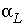= 0.015 m. The stagnant zone consists of spheres with radius a = 0.01 m, diffusion coefficient D e = 3.e-10 m 2 /s, and a shape factor= 0.21. This gives an exchange factor= 6.8e-6 s -1 . In the PHREEQC input file,, andmust be given; R m and R im are calculated implicitly by PHREEQC through the geochemical reactions.

Figure 4 shows the comparison of PHREEQC with the analytical solution (obtained with CXTFIT, version 2, Toride and others, 1995). The agreement is excellent for Cl - ( R = 1), but the simulation shows numerical dispersion for Na + ( R = 2). When the grid is made finer so that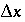is equal to or smaller than(0.015 m), numerical dispersion is much reduced. In the figure, the effect of a stagnant zone is to make the shape of the pulse asymmetrical. The leading edge is steeper than the trailing edge, where a slow release of chemical from the stagnant zone maintains higher concentrations for a longer period of time.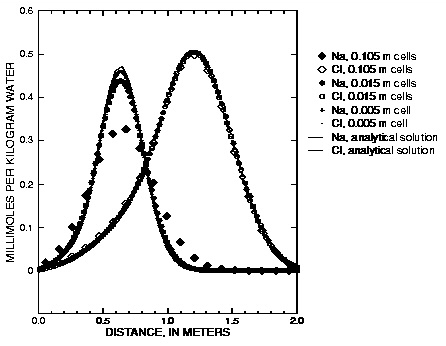Figure 4. --Analytical solution for transport with stagnant zones, a pulse input, and ion-exchange reactions compared with PHREEQC calculations at various grid spacings.

## Finite Differences for the Stagnant Zone

As an alternative to first-order exchange of stagnant and mobile zones, a finite difference grid can be laid over the stagnant region. Fick's diffusion equations,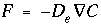and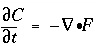, transform to finite differences for an arbitrarily shaped cell j :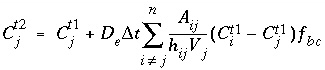, (126)

where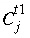is the concentration in cell j at the current time,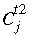is the concentration in cell j after the time step,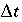is the time step [s, equal to () D in PHREEQC], i is an adjacent cell, A ij is shared surface area of cell i and j (m 2 ), h ij is the distance between midpoints of cells i and j (m), V j is the volume of cell j (m 3 ), and f bc is a factor for boundary cells (-). The summation is for all cells (up to n ) adjacent to j . When A ij and h ij are equal for all cells, a central difference algorithm is obtained that has second-order accuracy [ O ( h ) 2 ]. It is therefore advantageous to make the grid regular.

The correction factor f bc depends on the ratio of the volume of the mobile zone, V m , to the volume of the boundary cell which contacts the mobile zone, V bc . When the two volumes are equal, f bc = 1. It can be shown that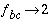when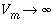(or if the concentration is constant in the mobile region, Appelo and Postma, 1993, p. 376). Likewise, f bc = 0 when V m = 0. To a good approximation therefore,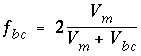. (127)

Equation 126 can be restated in terms of mixing factors for combinations of adjacent cells. For an adjacent cell, the mixing factor contains the terms which multiply the concentration difference ( C i - C j ),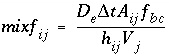(128)

and for the central cell, the mixing factor is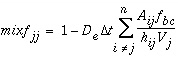, (129)

which give in equation 126: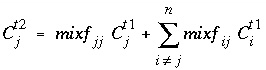. (130)

It is necessary that 0 < mixf < 1 to prevent numerical oscillations. If any mixf is outside the range, the grid of mobile and stagnant cells must be adapted. Generally, this requires a reduction of, which can be achieved by increasing the number of mobile cells. An example calculation is given in example 13, where the stagnant zone consists of spheres.

For data input to PHREEQC, 1D transport including only advection is accomplished with the ADVECTION data block. All other 1D transport calculations, including diffusion, advection and dispersion, and advection and dispersion in a dual porosity medium, require the TRANSPORT data block. Initial conditions of the transport column are defined with SOLUTION (or SOLUTION_SPREAD), EQUILIBRIUM_PHASES, EXCHANGE, GAS_PHASE, SOLID_SOLUTIONS, and SURFACE data blocks. Kinetic reactions are defined with KINETICS data blocks. Infilling solutions are defined with SOLUTION (or SOLUTION_SPREAD) data blocks (see "Description of Data Input").

| Next|| Previous || Top |